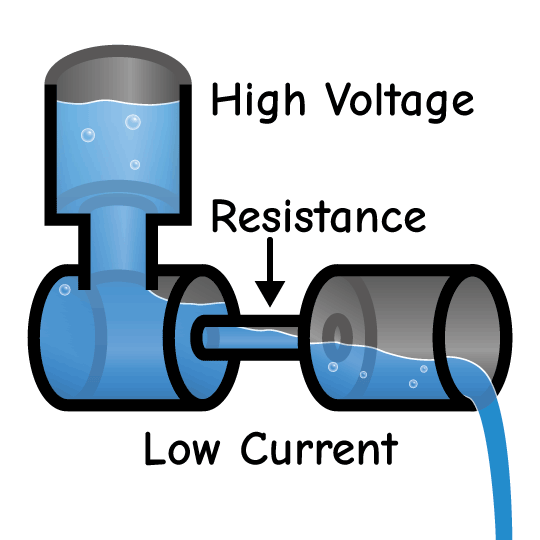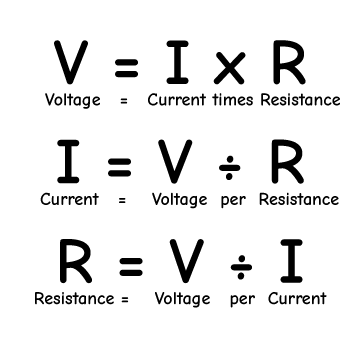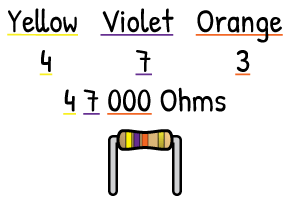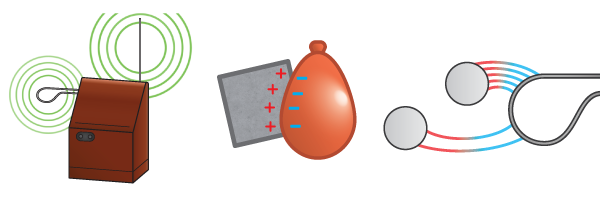# What's a Resistor?The most basic electrical part is the resistor. It restricts the flow of electricity. Just like a long narrow pipe will only let some water flow, a resistor will only let a certain amount of current flow for a given voltage.Inside resistors, electricity flows through a narrow thin film. The same way a long or narrow pipe restricts the flow of water, this film makes it difficult for electrons to travel. The longer or narrower the film inside the resistor, the more resistance.Resistance is measured in ohms: One volt is needed to create one amp of current through a one ohm resistor. Decreasing the resistance but keeping the voltage the same will increase the current. Keeping the resistance the same but increasing the voltage will increase the current.

Voltage (V) equals Current (I) times Resistance (R). This relationship between resistance, voltage, and current is called Ohm’s Law. There are three different ways to write Ohm’s Law depending on what you want to calculate.

You can use Ohm's Law to find how much voltage you will need to create a certain amount of current flow through a given resistance. Multiply the current and resistance to find the voltage.

Need to know how much current will flow through a resistor at a voltage? Divide voltage by resistance.

Need to pick a resistor that will create the right amount of current at a given voltage? Divide voltage by current to find how much resistance your resistor needs.The color bands around resistors are a code. Each color represents a number, and decoding them shows how many ohms the resistor has. The first two bands are the two biggest digits, and the third band shows the number of zeros that follow. The fourth golden band shows that the resistor has a tolerance, or accuracy, of 5%. Digikey has an online calculator for resistor color codes that you can visit by clicking here.

### Click on an Article Below to Keep Learning